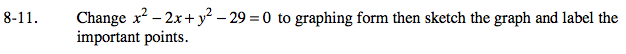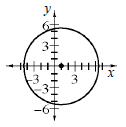Home > A2C > Chapter 8 > Lesson 8.1.1 > Problem8-11

8-11.

Change x2 − 2x + y2 − 29 = 0 to graphing form then sketch the graph and label the important points. Homework Help ✎Complete the squares for x and y.

Where is the center? Where are the x- and y-intercepts?